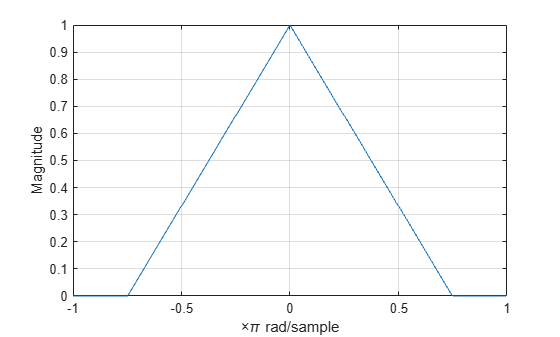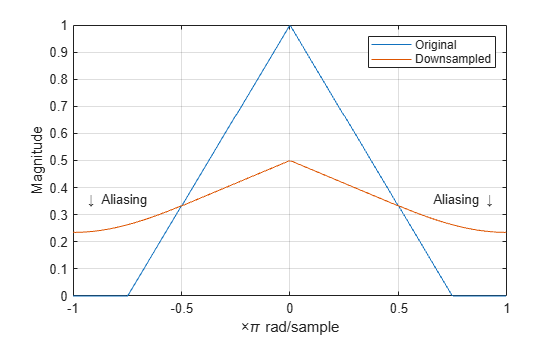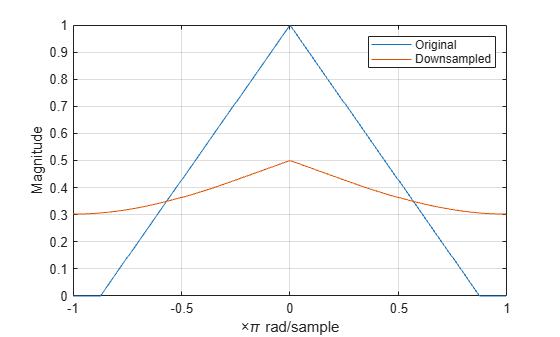## Downsampling — Aliasing

This example shows how to avoid aliasing when downsampling a signal. If a discrete-time signal's baseband spectral support is not limited to an interval of widthradians, downsampling byresults in aliasing. Aliasing is the distortion that occurs when overlapping copies of the signal's spectrum are added together. The more the signal's baseband spectral support exceedsradians, the more severe the aliasing. Demonstrate aliasing in a signal downsampled by two. The signal's baseband spectral support exceedradians in width.

Create a signal with baseband spectral support equal toradians. Use `fir2` to design the signal. Plot the signal's spectrum.

```F = [0 0.2500 0.5000 0.7500 1.0000]; A = [1.00 0.6667 0.3333 0 0]; Order = 511; B1 = fir2(Order,F,A); [Hx,W] = freqz(B1,1,8192,'whole'); Hx = [Hx(4098:end) ; Hx(1:4097)]; omega = -pi+(2*pi/8192):(2*pi)/8192:pi; plot(omega,abs(Hx)) xlim([-pi pi]) grid title('Magnitude Spectrum') xlabel('Radians/Sample') ylabel('Magnitude') ```You see that the signal's baseband spectral support exceeds.

Downsample the signal by a factor of 2 and plot the downsampled signal's spectrum with the spectrum of the original signal.

```y = downsample(B1,2,0); [Hy,W] = freqz(y,1,8192,'whole'); Hy = [Hy(4098:end) ; Hy(1:4097)]; hold on plot(omega,abs(Hy),'r','linewidth',2) legend('Original Signal','Downsampled Signal') text(-2.5,0.35,'\downarrow aliasing','HorizontalAlignment','center') text(2.5,0.35,'aliasing \downarrow','HorizontalAlignment','center') hold off ```In addition to an amplitude scaling of the spectrum, the superposition of overlapping spectral replicas causes distortion of the original spectrum for.

Increase the baseband spectral support of the signal toand downsample the signal by 2. Plot the original spectrum along with the spectrum of the downsampled signal.

```F = [0 0.2500 0.5000 0.7500 7/8 1.0000]; A = [1.00 0.7143 0.4286 0.1429 0 0]; Order = 511; B2 = fir2(Order,F,A); [Hx,W] = freqz(B2,1,8192,'whole'); Hx = [Hx(4098:end) ; Hx(1:4097)]; omega = -pi+(2*pi/8192):(2*pi)/8192:pi; plot(omega,abs(Hx)) xlim([-pi pi]) y = downsample(B2,2,0); [Hy,W] = freqz(y,1,8192,'whole'); Hy = [Hy(4098:end) ; Hy(1:4097)]; hold on plot(omega,abs(Hy),'r','linewidth',2) grid legend('Original Signal','Downsampled Signal') xlabel('Radians/Sample') ylabel('Magnitude') hold off ```The increased spectral width results in more pronounced aliasing in the spectrum of the downsampled signal because more signal energy is outside.

Finally, construct a signal with baseband spectral support limited to. Downsample the signal by a factor of 2 and plot the spectrum of the original and downsampled signals. The downsampled signal is full band, but the shape of the spectrum is preserved because the spectral copies do not overlap. There is no aliasing.

```F = [0 0.250 0.500 0.7500 1]; A = [1.0000 0.5000 0 0 0]; Order = 511; B3 = fir2(Order,F,A); [Hx,W] = freqz(B3,1,8192,'whole'); Hx = [Hx(4098:end) ; Hx(1:4097)]; omega = -pi+(2*pi/8192):(2*pi)/8192:pi; plot(omega,abs(Hx)) xlim([-pi pi]) y = downsample(B3,2,0); [Hy,W] = freqz(y,1,8192,'whole'); Hy = [Hy(4098:end) ; Hy(1:4097)]; plot(omega,abs(Hx)) hold on plot(omega,abs(Hy),'r','linewidth',2) grid legend('Original Signal','Downsampled Signal') xlabel('Radians/Sample') ylabel('Magnitude') hold off ```You see in the preceding figure that the shape of the spectrum is preserved. The spectrum of the downsampled signal is a stretched and scaled version of the original signal's spectrum, but there is no aliasing.